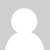### Reading sinusoidal wave with Arduino

Hi guys, before I start, I just want to say that I try searching but 'nothing' help me.

I live in Brazil, the voltage here is about 127V 60Hz, I bought an SCT-1250-400 for a project in my university, we will measure the consumption in building, the current is almost 300A more during a hot day.

What I did till now: The output of the CT is 0 to 333mV AC, where I know, I can't put this voltage directly in the Arduino analog port, and even if I could, the mV is not enough, so, I amplifier with a Amp op and sum with 2.5V, now I have a sinusoidal wave starting from 0 to 5V (the middle point is in 2.5V).

The big problem is, how can I read this with a Arduino, if the voltage in the analog port is between 0-5V, the current passing trough the CT is 400A, and the arduino should show me 400A in the serial monitor.

How can I get there?

### Re: Reading sinusoidal wave with Arduino

Everything you need to understand how to do this, and solve your problem, is in the section Building Blocks. The first page to read is "CT sensors - Interfacing with an Arduino", then you should download and understand the sketch "arduino-sketch-current-only" and the emonLib library. It is the library class "EnergyMonitor" and the method "EnergyMonitor::calcIrms( )" where the rms value of the voltage at the analogue input is calculated and multiplied by a scale factor to print the correct current. You will need to understand the calibration theory so that you can calculate and supply the correct calibration constant to the library in "EnergyMonitor::current(...)".### Re: Reading sinusoidal wave with Arduino

Thanks Robert, I'll try!### Re: Reading sinusoidal wave with Arduino

Robert,

I read the material but I couldn't find how to solution another problem. Here in Brazil, the voltage is 127V  (in this moment, my multimeter is showing 118V). I'm using a transformer 127V / 8V, I create and measuring every component, the board now look like the image attached.

Question 1: How can I make the calibration for this, I tried (127*11 / 8*1.2) but the value showing in the Serial Monitor from arduino is showing me 142V. I also tried (118*11/8*1.2) . I also would like to know why I multiply by 11. Just to know, I'm not using emontx.

Question 2: I don't understand the phase shift (emon1.voltage(2, 234.26, 1.7)), maybe that's my problem?

The Serial Monitor is showing me:

26516.41 24946.56 173.86 143.49 1.06
26517.78 24948.83 173.84 143.52 1.06
26602.12 25030.10 174.13 143.74 1.06

I put the same Voltage from A2 in A1 port, just to test, I'm trying just make the voltage work. If voltage work, I'm make my CT work.

Thanks

### Re: Reading sinusoidal wave with Arduino

Voltage: "11" is the inverse ratio of the voltage divider, in your case it is  (110 + 20) / 20 = 6.5

If you measured your transformer output at 8 V with 118 V input, then you use those numbers and you do not need the "1.2" (The 1.2 is because the adapter we use is 9 V out fully loaded, approx. 10.8 V unloaded, and we use it unloaded: 9 x 1.2 = 10.8).

Therefore your voltage calibration constant should be 118 x 6.5 / 8 = 95.875### Re: Reading sinusoidal wave with Arduino

Thanks Robert,

I did the calculus again, I measured 124V/8.3, the voltage calibration constante should be 97.11, I put it in the arduino sketch and made the upload again, but the voltage that appears in the Serial Monitor was almost 15V more than my multimeter was show me. So, I went down the value of the constant, when I stopped in 90.7 the Serial monitor shows me the exactly value of the multimeter (+/-0.3V of diference).

Should I left the constant iguals to 90.7 or found why the value of 97.11 make the diference about 15V ??

Thanks

### Re: Reading sinusoidal wave with Arduino

You should use the number that gives you the correct answer - always assuming your multimeter is correct! The difference is likely to be because the internal reference voltage of the ADC is not exactly 1.1 V - it has a 9% (IIRC) tolerance so that, added to the tolerance of your voltage divider resistors, accounts for the difference. http://openenergymonitor.org/emon/buildingblocks/emontx-error-sources tells you where the errors creep in.